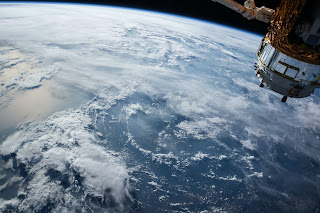### What is Geodetic Surveying? Explained With Video

The geodetic survey involves the survey of extensive areas that need to take into consideration the curvature of the earth, shape of earth, and size of the earth.So, in this method, the baseline measurement for performing triangulation ( Dividing the survey area into well-conditioned triangles for ease of computation) is measured from the seal level length and certain corrections are made for spherical excess in terms of angular values.

Watch Video: What is Geodetic Surveying?

Geodetic surveys are used when plane surveying cannot provided desired accuracy and precision. Now, geodetic surveys are performed by using satellites that are placed 12,500 miles away from the earth's surface.

### Features of Geodetic Surveying

The main features of geodetic survey are:

1. The two points are located so far that the earth's curvature cannot be neglected.

2. Plumb bob lines intersect at earth centre

3. Used to Survey Areas greater than 195.5sq.km

4. Used to Survey widely distant points and set them as control stations. Later precision points are surveyed using these control stations.

5. Angles Formed by Triangles exceed 180 degrees. This is called a spherical excess. The triangles formed in geodetic surveying are spherical triangles with arched sides.

### Objective of Geodetic Surveying

The main objectives of geodetic surveying are:

1. Determine the relative positions of points distant away with respect to the earth's surface.
2. To develop control points to conduct less precision survey works.
3. To develop reconnaissance information.

### Process of Geodetic Surveying

Geodetic surveying follows the general procedure:

1. Two station points that are at a considerable distance away are chosen.
2. The latitude and longitude of these points are determined astronomically.
3. The line joining the two points is called the baseline, which is measured accurately.
4. The position of a third point is determined by an angle made with each end of the baseline.
5. The process develops a triangle, and it is called triangulation. The procedure is continued till the entire survey land is mapped.

Watch Video on Geodetic Surveying Here>>